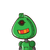# Are (2x)3 and 2×3 equivalent? In other words, is (2x)3 = 2×3? Explain.

Are (2x)3 and 2×3 equivalent? In other words, is (2x)3 = 2×3? Explain.

### 1 thought on “Are (2x)3 and 2×3 equivalent? In other words, is (2x)3 = 2×3? Explain.”

1.Step-by-step explanation:

No they are not equivalent value of (2x)³ is 8x³

so (2×³) is not equal to (2×)³